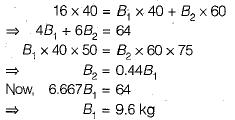# Balancing - 1

## 10 Questions MCQ Test Topicwise Question Bank for GATE Mechanical Engineering | Balancing - 1

Description
This mock test of Balancing - 1 for Mechanical Engineering helps you for every Mechanical Engineering entrance exam. This contains 10 Multiple Choice Questions for Mechanical Engineering Balancing - 1 (mcq) to study with solutions a complete question bank. The solved questions answers in this Balancing - 1 quiz give you a good mix of easy questions and tough questions. Mechanical Engineering students definitely take this Balancing - 1 exercise for a better result in the exam. You can find other Balancing - 1 extra questions, long questions & short questions for Mechanical Engineering on EduRev as well by searching above.
QUESTION: 1

### At a particular rotational speed, the unbalanced force due to revolving mass

Solution:

Magnitude = mω2r .
It direction is perpendicular to the perphering of rotational envelope in the same plane which changes at every angle.

QUESTION: 2

Solution:
QUESTION: 3

### Consider a 6 tonnes rotor of a steam turbine running at 8000 rpm out to the extent of 0.2 cm. The centrifugal force tending to lift the turbine from its foundations will be about

Solution: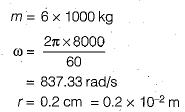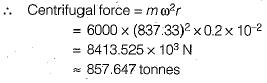QUESTION: 4

Consider a mass m attached at radius r from the axis of shaft which rotates with an angular velocity ω. The balancing is achieved by mounting a B kg mass at radius bfrom the axis of shaft. If the speed of shaft is doubled, then to attain perfect balance, the value of mass B should be

Solution: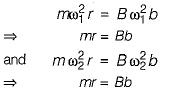∴ The value of balancing mass does not depend upon speed of rotation.

QUESTION: 5

For balancing a single disturbing mass, the minimum number of balance masses required to be introduced in a piane parallel to the plane of rotation of the disturbing mass will be

Solution: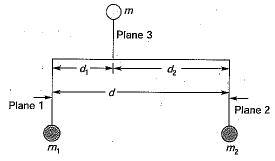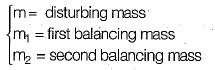QUESTION: 6

Which one of the following can completely balance several masses revolving in different planes on a shaft?

Solution:

Two balancing masses in different planes can completely balance several masses revolving in different planes.

QUESTION: 7

A system of masses rotating in different parallel planes is in dynamic balance if the resultant

Solution:

Condition for dynamic balancing ∑F = 0 and ∑M = 0.

QUESTION: 8

A system in dynamic balance implies that

Solution:
QUESTION: 9

Unbalanced force is produced due to eccentricity in the foliowing, except

Solution:
QUESTION: 10

if the planes of rotation of the three masses are parallel, then the balance mass B1 is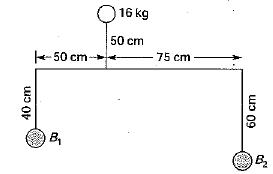Solution: